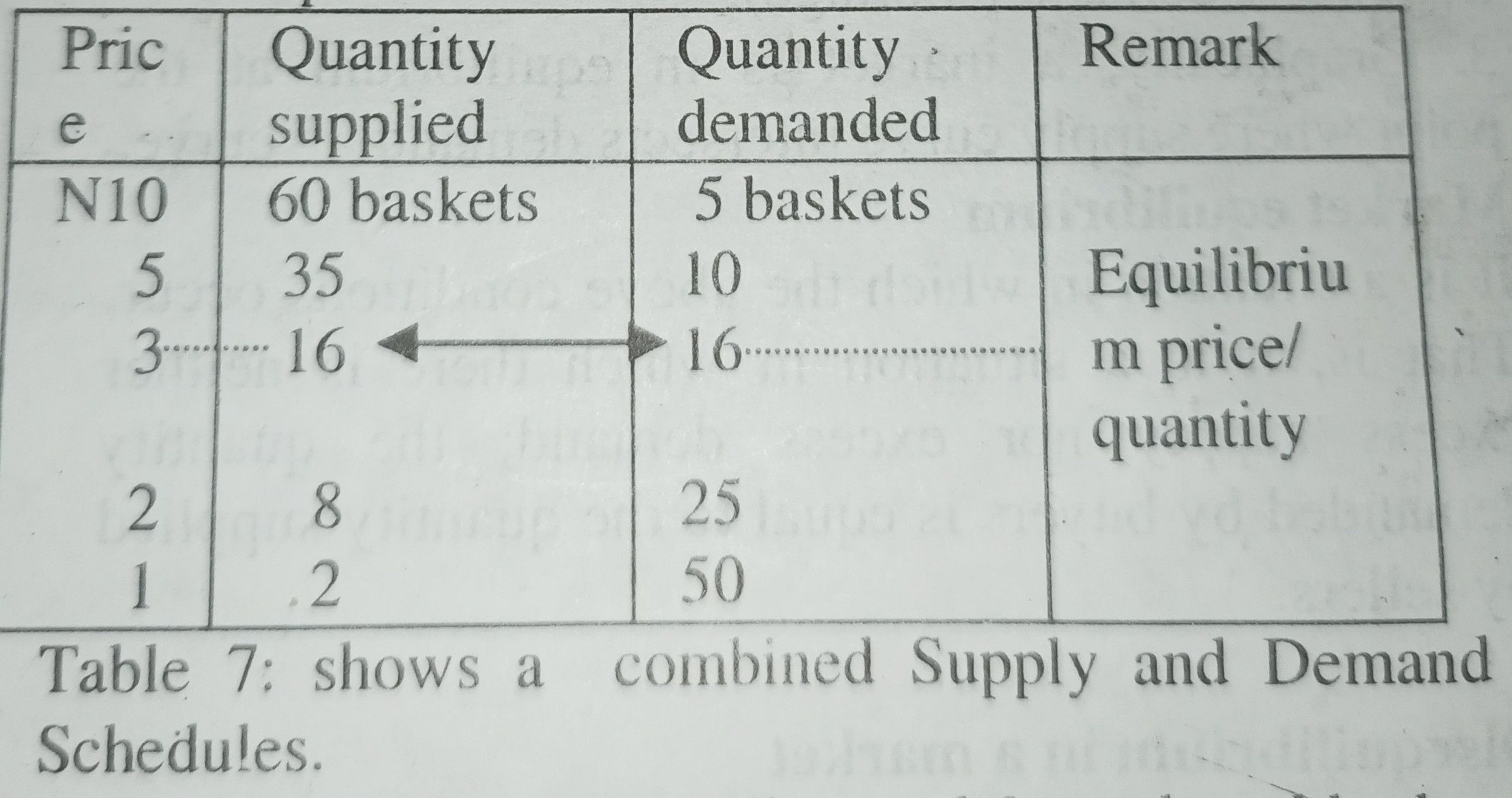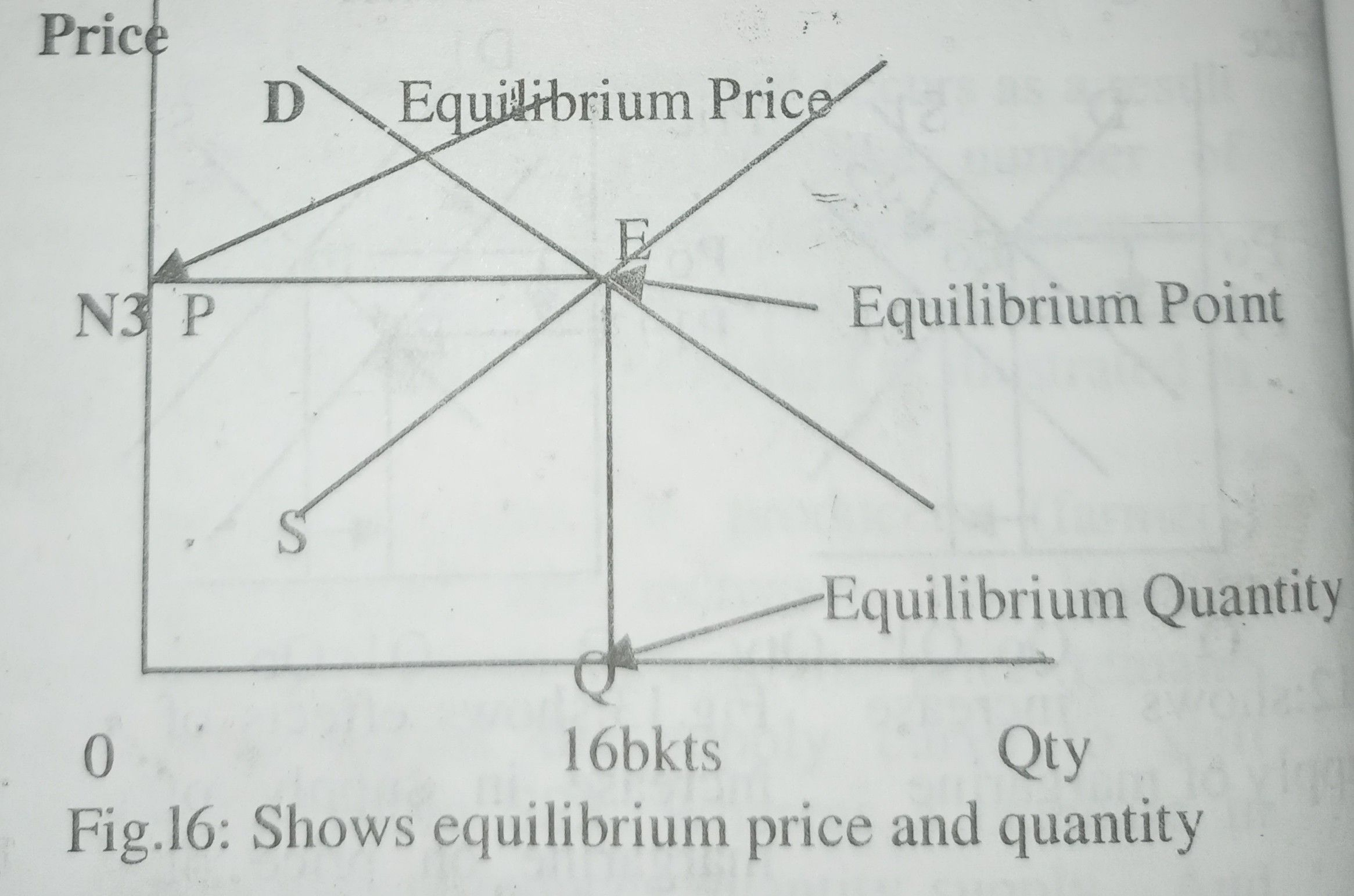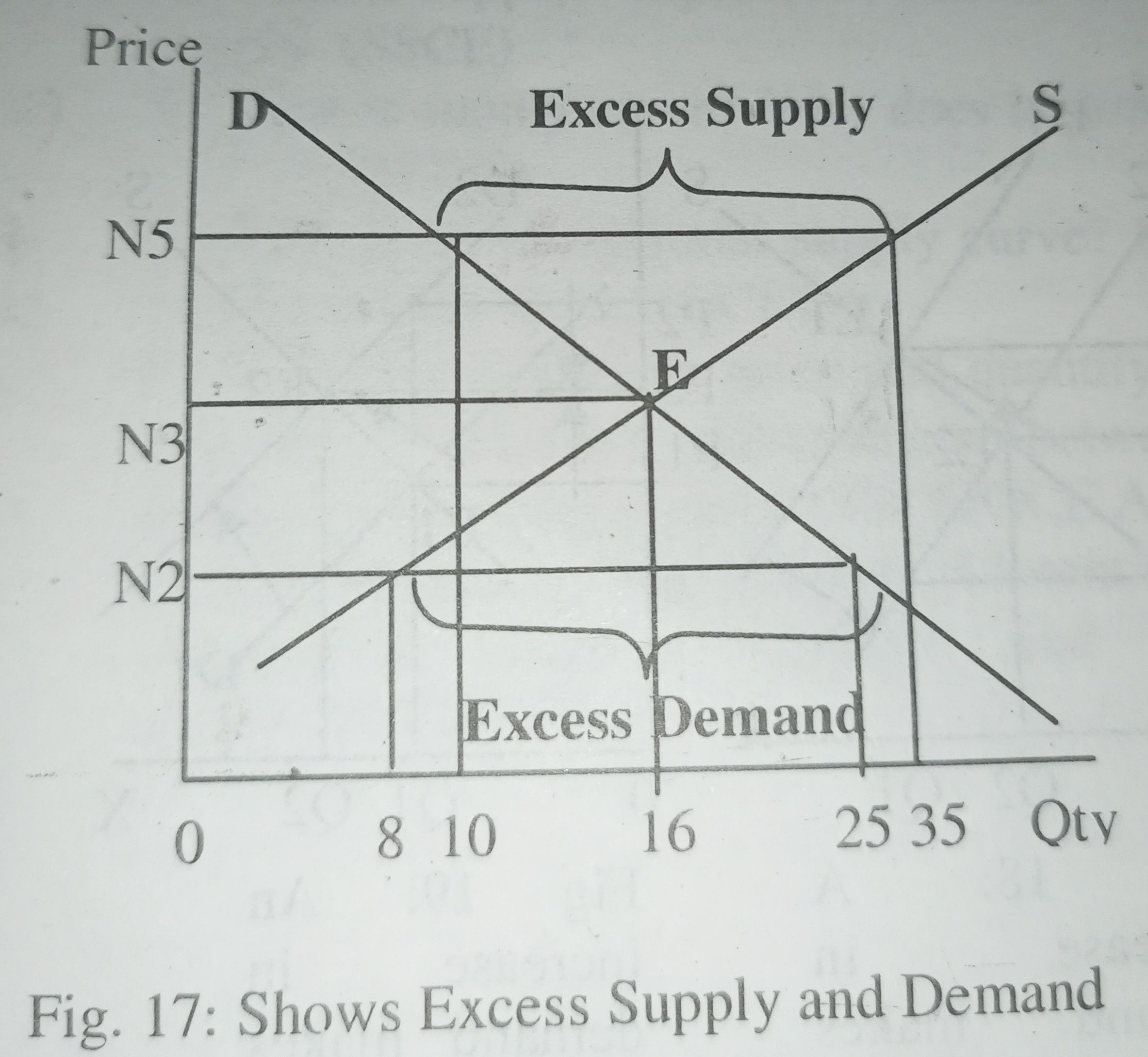Home » EQUILIBRIUM IN A MARKET

# EQUILIBRIUM IN A MARKETDetermination of equilibrium price and quantity in a market

Equilibrium in a market

Equilibrium means a state of balance of opposing forces. A market is in equilibrium:

1) When the total quantity of goods put forth for sale is equal to the total quantity of goods demanded. Briefly, it occurs when:

• desired sales = desired purchases.
• or quantity supplied = quantity demanded.

2) When there is neither excess supply (surplus) nor excess demand (shortage).

3) Graphically, a market is in equilibrium at the point where supply curve intersects demand curve.

Market equilibrium

It is a situation in which the above conditions occur. That is, it is a situation in which there is neither excess supply nor excess demand; the quantity demanded by buyers is equal to the quantity supplied by sellers.

Disequilibrium in a market

A market is in a state of disequilibrium if the above market conditions do not hold. In other words, disequilibrium occurs in the process of sales and purchases (in a market) if the following occur:-:

1. If the total quantity supplied is not equal to the total quantity demanded.
2. If there is either excess supply (commodity glut – surplus) or excess demand (acute commodity shortage). That is, ‘increase or decrease in supply without a corresponding increase or decrease in demand.’ Either of the above conditions can cause disequilibrium in a market.

Restoring equilibrium in a market

An equilibrium is restored by rectifying (correcting) the above abnormal market conditions. That is, a state of equilibrium is achieved by:

1. Eliminating (removing) both excess ‘demand and excess supply.
2. Making increase or decrease in demand to correspond with (to be equal to) increase or decrease in supply.

Price determination

In a certain economic system, prices in a market are determined (fixed) by the forces of supply and demand. Where the two forces are equal sets the price. That is, where the quantity supplied equals to the quantity demanded determines the price. And such a price is called ‘Equilibrium price‘.

It is important to note, however, that in certain circumstances or situations like in planned economy, prices of certain items or essential goods may be determined by the government in form of price control. We ignore this system of fixing prices by the government and concentrate on determining prices by market forces of supply and demand.

Combination of demand and supply schedule data table.

A combined demand and supply schedule is both demand schedule and supply schedule brought together in one table. It shows the different quantities of a commodity (onion) demanded and supplied at different prices.You might have observed from the table that at N3, the quantity demanded and supplied are equal. Such a price, N3.00, at which demand is equal to supply is called Equilibrium Price. And the quantity, 16 baskets, at which demand and supply are equal is called Equilibrium quantity.

Equilibrium Price

Equilibrium price is a price at which:

1) Demand is equal to supply: i.e. the quantity demanded is equal to quantity supplied.

Demand = Supply

Or forces of demand = forces of supply

2) Demand curve intersects supply curve. That is, it is the price that corresponds to the point of intersection of demand curve and supply curve. Equilibrium price is further illustrated graphically in figure below.Demand curve and supply curve intersects at point E – the equilibrium point. At this point, the quantity OQ is supplied and the same quantity is demanded and bought. And the price charged is OP – N3. At this price where there is neither shortage nor surplus of the commodity in the market is called Equilibrium price.

Equilibrium Quantity

1) It is the quantity at which demand is equal to Supply:

Demand = Supply

2) It is the quantity at which demand curve intersects supply curve. That is, it is the quantity that corresponds with the point where demand curve intersects supply curve.

Graphically, equilibrium quantity, OQ, lies on the horizontal axis. While equilibrium price lies on the vertical axis as indicated in figure 16. In the above table 7, equilibrium price is N3.00 and equilibrium quantity is 16 baskets of onion. It is important to note, however, that if control price is more than equilibrium price, supply rises above the equilibrium level.

It is said that ‘price moves toward the point where demand is equal to supply‘. This is a situation of a free enterprise economy or market economy where prices are determined by the free interplay of market forces of supply and demand.

At a particular price, say N5, the quantity supplied is 35 units; while the quantity demanded is 10 units. There is excess supply of 25 units. This makes the price to fall (move downward).

At a low price, say N2 per unit, the demand rises to 25 units; but supply drops to 8 units. This causes excess demand of 17 units. This makes the price to rise (move upward).

From the above, excess supply brings down the price. While excess demand causes a rise in price. These situations continue until the price moves to a level, N3, where there is neither excess supply nor excess demand. Demand and supply are equal at this price level of N3. And there is no tendency for the price to change. The equilibrium price and quantity are therefore N3 and 16 baskets respectively.

Third law of supply and demand

We recall that equilibrium price is the price where the forces of supply and demand come into contact. The third law of supply and demand therefore states that

“Price in a perfect market is determined by the interaction of the two forces of supply and demand, the intensity of demand in relation to the conditions of supply”.

Other related concepts (other relevant terms)

Excess demand

It occurs when the quantity demanded is more than the quantity supplied. It is the quantity, by which demand exceeds supply. Thus it is obtained by subtracting quantity supplied from quantity demanded.From the above combined table 7, excess demand at N2.00 is 25 – 8 = 17 baskets

Excess Supply

It occurs when quantity supplied is more than quantity demanded. “It is the quantity by which supply exceeds demand.” Thus it is obtained by subtracting quantity demanded from quantity supplied. And from the above table 7, excess supply at N5 is: 35 – 10 = 25 baskets.

And we wish to illustrate excess demand and supply with a means of a diagram as illustrated in the figure below.Note:

Excess supply always occurs above the equilibrium point – point E; while excess demand occurs below the equilibrium point. If control price is above the equilibrium price, supply rises above equilibrium supply.

Summary

• Equilibrium price = N3.
• Equilibrium quantity = 16 baskets.
• Excess supply occurs at N5 and N10.
• Excess demand occurs at N1 and N2
• Excess supply at N5 =35 – 10 = 25 baskets.
• Excess demand at N2 = 25 – 8 = 17 baskets.